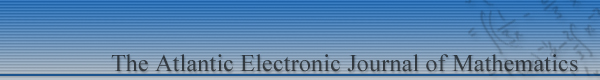Home News Aims and Scope Information for Authors Editorial Board Browse AEJM online ContactVolume 5
Summer 2012
Number 1

## Quadrature rules with (not too many) derivatives

#### Matthew Wiersma

Abstract: Quadrature formulas for $\int_a^b f(x) dx$ where derivative terms need only be evaluated at $a$ and $b$ in the composite rule are identified. Error bounds are given when $f:[a,b]\to\R$ satisfies $f^{(n-1)}$ is absolutely continuous so that $f^{(n)}\in L^p([a,b])$, and when $f^{(n-1)}$ is merely continuous.